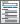Home  /  Products  /  Features  /  Latent class analysis (LCA)

## Latent class analysis (LCA)

Discover and understand unobserved groups (latent classes) in your data–whether the groups are consumers with different buying preferences, healthy and unhealthy individuals, or teens with high, medium, and low risk of high school drop out. You can use LCA as a model-based method of classification. Or you can fit SEM path models and test for differences across the unobserved groups. Estimate the proportion of the population in each group, estimate group means, and more.

Categorical latent variables measured by• Binary items
• Ordinal items
• Continuous items
• Count items
• Categorical items
• Fractional items
• Survival times

Model class membership• Predictors of class membership
• Multinomial logistic model

Starting values

• EM algorithm
• Fixed or random starting values
• Select number of random draws
• Easily specify equality constraints across classes
• Constrain one parameter
• Cross-class equality constraints—just type lcinvariant(cons) to constrain intercepts

Multiple-group models• Allow for differences in LCA across known groups
• Group estimation is as easy as group(agegroup)
• Some parameters constrained and others estimated freely across groups

Goodness of fit• Likelihood-ratio test vs saturated model (G2 statistic)
• AIC
• BIC

Inferences

• Class membership
• Posterior class membership
• Predicted means, probabilities, counts
• For each latent class
• Marginal with respect to latent classes
• Marginal with respect to posterior latent classes
• Survivor function
• Density function
• Distribution function

Postestimation Selector

• View and run all postestimation features for your command
• Automatically updated as estimation commands are run

Factor variables

• Automatically create indicators based on categorical variables
• Form interactions among discrete and continuous variables
• Include polynomial terms
• Perform contrasts of categories/levels

Marginal analysis

• Estimated marginal means
• Marginal and partial effects
• Average marginal and partial effects
• Adjusted predictions, means, and effects
• Works with multiple outcomes simultaneously
• Contrasts of margins
• Pairwise comparisons of margins
• Profile plots
• Graphs of margins and marginal effects